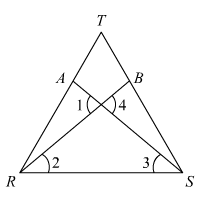#### Chapter 10 Congruent Triangles R.D. Sharma Solutions for Class 9th Exercise 10.2

Exercise 10.2

1. In Fig. 10.40, it is given that RT = TS, ∠1 = 2∠2 and ∠4 = 2∠3. Prove that ΔRBT ≅ΔSAT.Solution2. Two lines AB and CD intersect at O such that BC is equal and parallel to AD. Prove that the lines AB and CD bisect at O.

Solution3.  BD and CE are bisectors of ∠B and ∠C of an isosceles ΔABC with AB = AC. Prove that BD = CE.

Solution